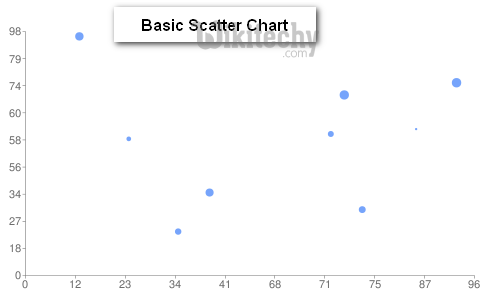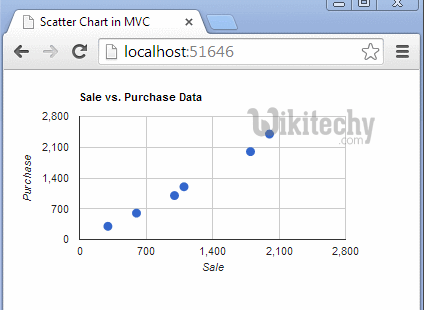## What is Basic Scatter Chart?

• Basic Scatter Chart data is displayed as a collection of points where one variable determines the position on the horizontal axis whereas the other variable determines the position on the vertical axis.
• Basic Scatter Chart is useful when we wish to see how two comparable data sets agree to show nonlinear relationships between the variables.
• Basic Scatter Chart offers a range of displays of paired combinations of categorical and quantitative variables.
• Basic Scatter Chart enables the researcher to obtain a visual comparison of the two variables in the data set, and will determine the relationship between two variables.
• Basic Scatter Chart has an equation for the correlation between the variables which can be determined by establishing best-fit procedures## Configuration

• The syntax which is given below gives us the configuration of Basic Scatter Chart and we have added Scatter Chart class to show scatter based chart

## Syntax:

• The sample code which is given below show us the full sample code of basic scatter chart